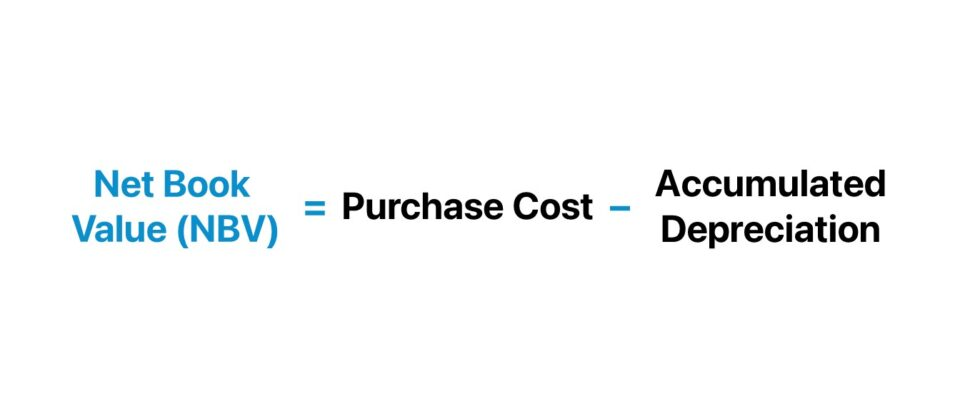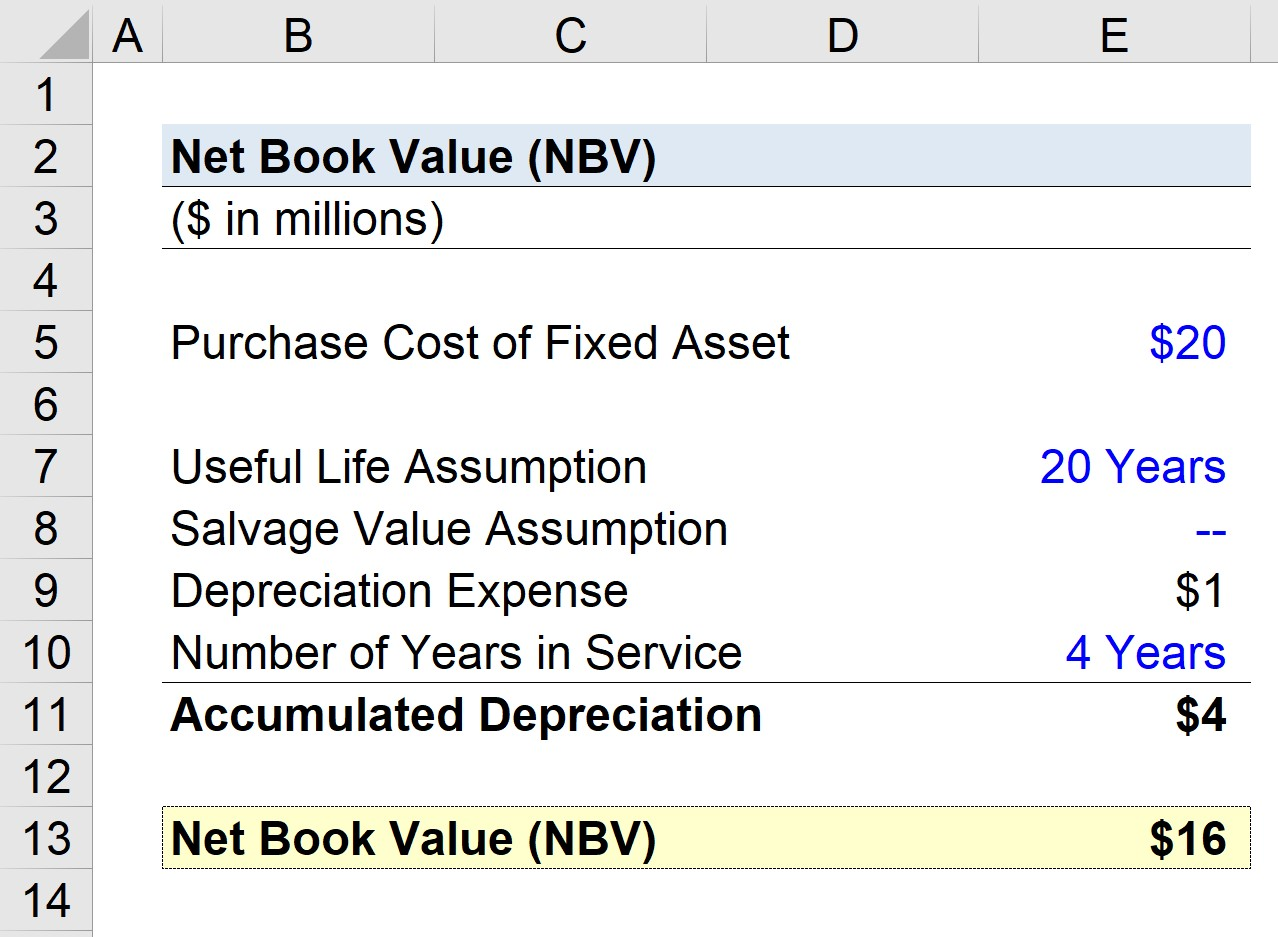Welcome to Wall Street Prep! Use code at checkout for 15% off.# Net Book Value (NBV)

Guide to Understanding Net Book Value (NBV)• The net book value (NBV) is the carrying value of an asset recorded on a company’s balance sheet for bookkeeping purposes.
• The net book value (NBV) formula subtracts the purchase cost of a fixed asset (PP&E) by its accumulated depreciation to date.
• The net book value (NBV) is important because the purchase of fixed assets (PP&E) – or capital expenditures (Capex) – must be capitalized on the balance sheet since their useful life assumption exceeds twelve months per accrual accounting standards.
• The net book value (NBV) is different from the market value as NBV represents the historical cost of an asset, which is then reduced by accumulated depreciation, amortization, asset impairment, and asset write-down.

## How to Calculate Net Book Value (NBV)?

NBV stands for “Net Book Value” and refers to the carrying value of an asset recognized on the balance sheet of a company, prepared for bookkeeping purposes.

The starting point for calculating an asset’s net book value (NBV) is its historical cost, which refers to the purchase cost of the fixed asset (PP&E).

Under accrual accounting reporting standards – specifically, the historical cost principle – the value of a long-term, fixed asset belonging to a company is recognized as the purchase cost as of the date of original purchase.

The net book value (NBV) is most applicable to fixed assets (PP&E), which must be capitalized on the balance sheet since their useful life assumption is expected to exceed twelve months.

Otherwise, the short-term asset with a useful life less than twelve months, such as accounts receivable (A/R) and inventory, is recognized in the current assets section of the balance sheet.

The concept of depreciation in accrual accounting reduces the fixed asset’s net book value (NBV) in accordance with its useful life and salvage value assumption to match the recognition of the expense with the period in which the economic benefit is received.

• Income Statement (I/S) → The depreciation expense is embedded within the cost of goods sold (COGS) and operating expenses (Opex) section, and reduces the reported net income (the “bottom line”) of a company.
• Cash Flow Statement (CFS) → Since depreciation is a non-cash expense, in which the real outflow of cash occurred on the date of purchase, the depreciation expense is treated as an add-back on the cash flow statement (CFS)/
• Balance Sheet (B/S) → On the balance sheet, the annual depreciation expense reduces the recorded balance of the fixed asset (PP&E) over the course of its useful life.

Based on the specific fixed asset in question, the historical cost of an asset can be reduced by the following factors.

## Net Book Value Formula (NBV)

The formula for calculating the net book value (NBV) of a fixed asset (PP&E) is as follows.

Net Book Value (NBV) = Purchase Cost of Fixed Asset Accumulated Depreciation

Where:

• Purchase Cost of Fixed Asset → The incurred capital expenditure (Capex) from the purchase of the long-term, fixed asset.
• Accumulated Depreciation → The total depreciation recognized to reduce the value of the purchased fixed asset to date.

While only the accumulated depreciation is deduced from the purchase cost here, the formula can become more complex if there are other additional variables, such as if the company determines that the fixed asset is impaired and must be written down on the books.

Impairment stems from a circumstance wherein the company decides that an asset’s market value is less than its net book value, i.e. a downward reduction is applied to the asset’s book value to reflect its true value more accurately.

In effect, the carrying value of a fixed asset (PP&E) is gradually reduced, however, the stated amount on the balance sheet does not reflect its fair value as of the present date.

## NBV vs. FMV: What is the Difference?

So, what is the difference between the net book value (NBV) and fair market value (FMV) of an asset?

• Net Book Value (NBV) → The net book value (NBV) reflected on a company’s balance sheet is seldom equal to, or even near, the market value of equity. Barring unusual circumstances, a company’s market value of equity – i.e. the market capitalization (“market cap”) – is most often substantially greater than the book value of equity reported on the balance sheet.
• Fair Market Value (FMV) → Unlike the net book value (NBV), the fair market value (FMV) of a company’s equity is adjusted to reflect the fluctuations in value based on the current state of the market conditions and investor sentiment as of the present date, rather than on the original date of purchase and conservative accounting adjustments. Likewise, the same concept is applied to the value assigned to the fixed assets recorded on a company’s balance sheet.

Hence, the net book value (NBV) is not equivalent to its fair market value (FMV) because the former is intended for bookkeeping purposes, while the latter is meant to reflect the current value.

## Net Book Value Calculator (NBV)

We’ll now move to a modeling exercise, which you can access by filling out the form below.Submitting...

## 1. Fixed Asset (PP&E) Assumptions

Suppose a company is estimating the net book value (NBV) of a fixed asset (PP&E) to record on its balance sheet. The original purchase price associated with acquiring the fixed asset – i.e. the capital expenditure (Capex) – was \$20 million.

• Purchase Cost of PP&E = \$20 million

With regard to the assumptions surrounding the fixed asset, the useful life assumption is 20 years, while the salvage value is assumed to be zero.

• Useful Life = 20 Years
• Salvage Value = \$0

## 2. Purchase Cost and Accumulated Depreciation Calculation Example

The annual depreciation expense equals the purchase cost of the fixed asset (PP&E), net of the salvage value, divided by the useful life assumption.

• Annual Depreciation Expense = (\$20 million – \$0 million) ÷ 20 Years = \$1 million

Given the above assumptions, what is the recorded net book value (NBV) in Year 4?

Since four years have passed, whereby the annual depreciation expense is \$1 million, the accumulated depreciation totals \$4 million.

• Number of Years in Service = 4 Years
• Accumulated Depreciation = \$1 million × 4 Years = \$4 million

## 3. Net Book Value Calculation Example (NBV)

If we subtract the \$4 million in accumulated depreciation from the fixed asset’s original purchase cost of \$20 million, we arrive at a net book value (NBV) of \$16 million.

• Net Book Value (NBV) = \$20 million – \$4 million = \$16 million

In conclusion, the \$16 million in net book value (NBV) reflects the ending balance of the fixed asset (PP&E) recorded on the balance sheet of the current period per the accrual accounting reporting standards established under US GAAP.Step-by-Step Online Course

### Everything You Need To Master Financial Modeling

Enroll in The Premium Package: Learn Financial Statement Modeling, DCF, M&A, LBO and Comps. The same training program used at top investment banks.